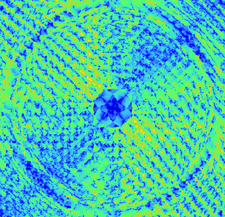issue contentsFOUNDATIONSADVANCES
ISSN: 2053-2733

November 2021 issueCover illustration: The exploration of novel magnetic materials requires characterization methods capable of displaying atomic scale behaviour. The multislice method is used in the simulation of high-resolution images in transmission electron microscopy; however the inclusion of magnetic effects in this method is challenging, particularly for larger systems. Lyon & Rusz [Acta Cryst. (2021). A77, 509–518] present a parameterized magnetism approach which is able to describe the effects of magnetism in large crystals to a good degree of accuracy. The cover image shows the logarithm of the relative ratio of the squared amplitude of the DFT and parameterized magnetism exit wavefunctions for body-centred cubic Fe.

research papers

Parameterization of magnetic vector potentials and fields for efficient multislice calculations of elastic electron scattering

A tabulation is presented of parameterized magnetic fields computed from atomic density functional theory calculations that allows for the efficient computation of approximate magnetic vector fields in materials using only structural and magnetic moment size and direction information. Multislice calculations of the change in the intensity of diffraction patterns due to magnetism for body-centred cubic Fe and FePt show that this approach is able to describe the effects of magnetism in these kinds of systems to a good degree of accuracy.

Vanishing of the atomic form factor derivatives in non-spherical structural refinement – a key approximation scrutinized in the case of Hirshfeld atom refinement

The approximation used in non-spherical refinement that the derivatives of the form factors are zero is investigated.

research papers

The role of an objective function in the mathematical modelling of wide-angle X-ray diffraction curves of semi-crystalline polymers

The choice of an objective function in constructing a mathematical model of an experimental wide-angle X-ray diffraction curve of semi-crystalline polymers is discussed.

Combinatorial aspects of the Löwenstein avoidance rule. Part I: the independence polynomial

Practical issues concerning the calculation of independence ratios of finite graphs are analyzed.

Layer groups: Brillouin-zone and crystallographic databases on the Bilbao Crystallographic Server

The set of databases of the Bilbao Crystallographic Server (https://www.cryst.ehu.es/) including crystallographic data on generators, general positions, Wyckoff positions, maximal subgroups and Brillouin-zone figures and k-vector tables for all 80 layer groups are discussed in detail and illustrated.

The wavevector star channel and symmetry group

The concepts of `wavevector star channel' and `wavevector star channel group' are newly proposed, which allow the effective study of phase transitions by considering directly the translational symmetry breaking in crystals. It is shown that the complicated symmetry changes in the pyroelectric crystal Pb1−xCaxTiO3 (PCT) can be described using the new five-component reducible order parameter transformed according to the representation of the wavevector star channel group but not the nine-component one based on the theory of the full irreducible representation of the space group.

X-ray molecular orbital analysis. II. Application to diformohydrazide, (NHCHO)2

The molecular orbitals of diformohydrazide were determined by X-ray molecular orbital analysis and it became evident that the molecular orbitals can be obtained by optimizing the electron density observed by X-ray diffraction.

On single-crystal total scattering data reduction and correction protocols for analysis in direct space

Data reduction and processing as related to single-crystal diffuse scattering and 3D-ΔPDF experiments are explored.